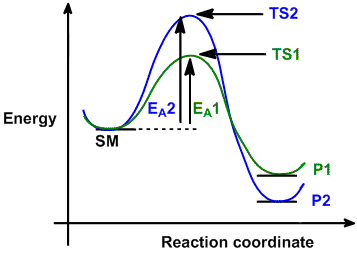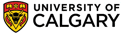Chapter 10: Conjugation in Alkadienes and Allylic SystemsKinetic and Thermodynamic Control

The potential outcome of a reaction is usually influenced by two factors:

1. the relative stability of the products  (i.e. thermodynamic factors)
2. the rate of product formation  (i.e. kinetic factors)
The following simple reaction coordinate diagram provides a basis for the key issues about kinetic and thermodynamic control:

 Consider the case where a starting material, SM, can react in a similar manner to give two different products, P1 and P2 via different (competing) pathways represented by green and blue curves.  Reaction 1 via pathway 1 (green)  generates product 1 (P1) via transition state 1 (TS1). This will be the faster reaction since it has a lower energy (more stable) transition state, and therefore a lower activation barrier. Therefore, product 1, P1 is the kinetic product (the product that forms the fastest). Reaction 2 via pathway 2 (blue) generates product 2 (P2) via transition state 2 (TS2). P2 is the more stable product since P2 is at a lower energy than P1. Therefore, P2 is the thermodynamic product (the more stable product).We now need to consider how the outcome of this situation changes with the competing reactions of the starting material as we alter the reaction temperature and therefore the average energy of the molecules changes.

1.  At low temperature, the average energy of the molecules is low and more molecules have enough sufficient energy cross activation energy EA1 than EA2. Therefore the reaction preferentially proceeds along the green path to P1. The reaction is not reversible since the molecules lack sufficient energy to reverse to SMi.e. it is irreversible, so the product ratio of the reaction is dictated by the rates of formation of P1 and P2, k1: k2.

2. At some slightly higher temperature, reaction 1 will become reversible when sufficient molecules have enough energy to cross the reverse reaction barrier for reaction 1, while reaction 2 remains irreversible. So although P1 may form initially, over time it will revert to SM and react to give the more stable P2.

3. At high temperature, both reaction 1 and 2 are reversible and the product ratio of the reaction is dictated by the equilibrium constants for P1 and P2, K1 : K2.

Summary

• At low temperature, the reaction is under kinetic control (rate, irreversible conditions) and the major product is that from the fastest reaction.
• At high temperature, the reaction is under thermodynamic control (equilibrium, reversible conditions) and the major product is the more stable system© Dr. Ian Hunt, Department of Chemistry